## Example Questions

### Example Question #61 : Arithmetic

Which of the following is equivalent to the ratio of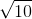to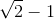?

Possible Answers: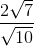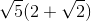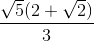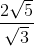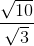Correct answer:Explanation:

At first, this problem seems rather easy. You merely need to divide these two values to get: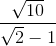However, there are no answers that look like this! When this happens, you should consider rationalizing the denominator to eliminate the square root. This is a little more difficult than normal problems like this (ones that contain only the radical). However, if you complete a difference of squares in the denominator, you will be well on your way to having the right answer: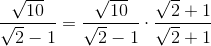Carefully FOIL your denominator and distribute your numerator: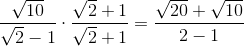Look to your answers for an idea for factoring your numerator: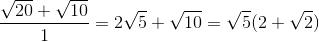### Example Question #62 : Arithmetic

What isif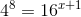?

Possible Answers:Correct answer:Explanation:

Note that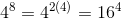This changes the initial equation to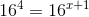For this equation to be valid, the exponents must be equal: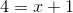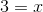### Example Question #63 : Arithmetic

Given the equation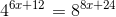, solve for.

Possible Answers: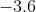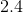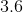Correct answer:Explanation:

In order to solve for, the equationmust be written such that each set of exponents shares the same base: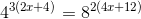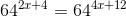Which like bases, it's now just a matter of solving for: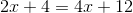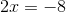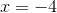### Example Question #64 : Arithmetic

Which of the following is equal to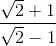?

Possible Answers: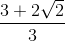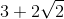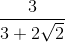Correct answer:Explanation:

To find an equivalent, just multiply the top and bottom by the conjugate of the denominator.

Conjugate is the square root expression found in the denominator but with opposite sign.

So: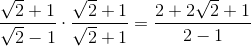By simplifying, we get.

### Example Question #65 : Arithmetic

Which of the following has the same ratio as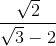?

Possible Answers: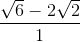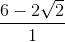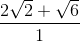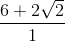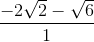Correct answer:Explanation:

Since in all the answer choices have an integer in the denominator, we should multiply top and bottom by the conjugate of the denominator which is the square root expression with opposite sign.

So: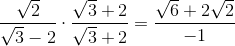If we redistribute the negative, then the answer becomes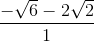.

### Example Question #66 : Arithmetic

What is the ratio of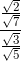expressed in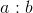form?

Possible Answers: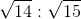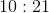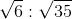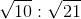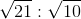Correct answer:Explanation:

To get intoform, multiply the fraction by bottom denominator's reciprocal.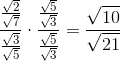Theis the numerator of the fraction andis the denominator.

Final answer is### All GRE Math Resources